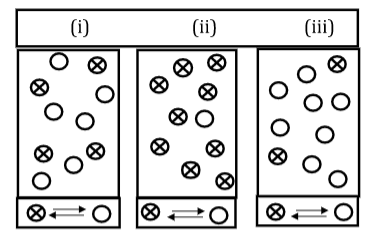Chemistry Practice Problems Equilibrium Expressions Practice Problems Solution: Each of the following diagrams represents a system...

🤓 Based on our data, we think this question is relevant for Professor All professors' class at GS.

# Solution: Each of the following diagrams represents a system at equilibrium at three different temperatures. Rank the three diagrams in order of increasing value for K (assume all chemicals are in gas phase). A) (i) &lt; (ii) &lt; (iii) B) (ii) &lt; (iii) &lt; (i) C) (iii) &lt; (ii) &lt; (i) D) (iii) &lt; (i) &lt; (ii) E) (ii) &lt; (i) &lt; (iii)

###### Problem

Each of the following diagrams represents a system at equilibrium at three different temperatures. Rank the three diagrams in order of increasing value for K (assume all chemicals are in gas phase).

A) (i) < (ii) < (iii)

B) (ii) < (iii) < (i)

C) (iii) < (ii) < (i)

D) (iii) < (i) < (ii)

E) (ii) < (i) < (iii)Equilibrium Expressions

Equilibrium Expressions

#### Q. A sample of pure carbon dioxide at 500 K undergoes spontaneous decomposition to produce the following reaction:2 CO2 (g) ⇌ 2 CO (g)  +  O2 (g)The equi...

Solved • Thu Sep 22 2016 12:52:25 GMT-0400 (EDT)

Equilibrium Expressions

#### Q. Express the equilibrium constant for the following reaction.P4O10 (s) ⇌ P4 (s) + 5 O2 (g)A) K = [P4][O2]5 / [P4O10]B) K = [P4O10] / [P4][O2]5C) K = [O...

Solved • Fri Sep 16 2016 12:30:35 GMT-0400 (EDT)

Equilibrium Expressions

#### Q. Consider the following reaction, equilibrium concentrations, and equilibrium constant at a particular temperature. Determine the equilibrium pressure ...

Solved • Fri Aug 26 2016 10:02:44 GMT-0400 (EDT)

Equilibrium Expressions

#### Q. Determine the value of Kc for the following reaction if the equilibrium concentrations are as follows:[N2]eq = 3.6 M, [O2]eq = 4.1 M, [N2O]eq = 3.3 x ...

Solved • Fri Aug 26 2016 09:46:17 GMT-0400 (EDT)